# Shading Fractions, Decimals and Percentages (2)

In this worksheet, students identify the fraction, decimal or percentage shaded in a rectangle.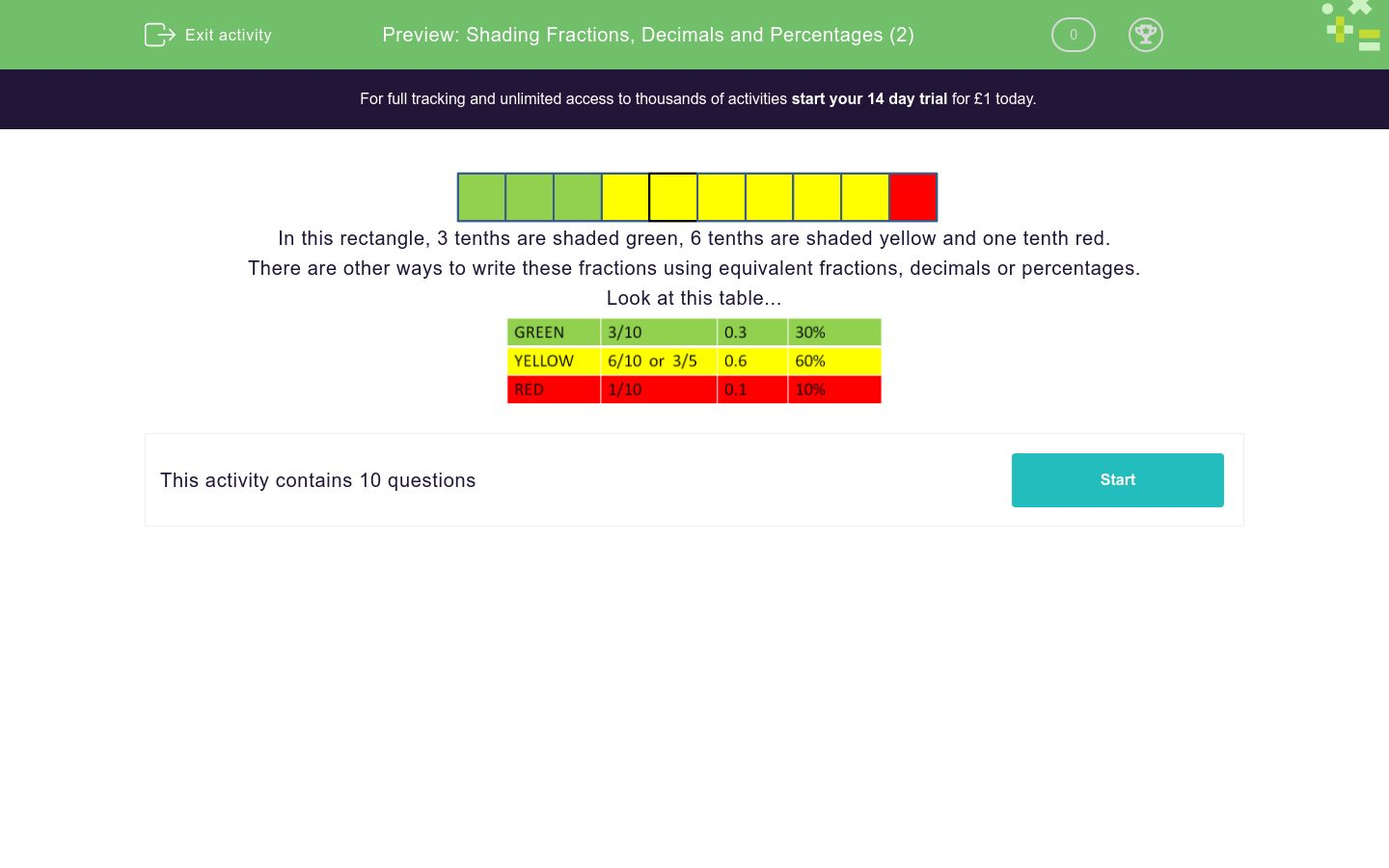Key stage:  KS 2

Curriculum topic:  Number: Fractions, Decimals and Percentages

Curriculum subtopic:  Know Fraction-Decimal-Percentage Equivalents

Difficulty level:### QUESTION 1 of 10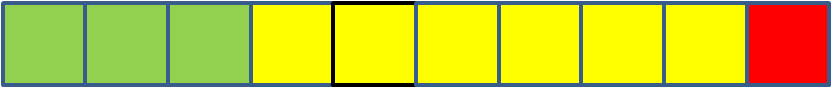In this rectangle, 3 tenths are shaded green, 6 tenths are shaded yellow and one tenth red.

There are other ways to write these fractions using equivalent fractions, decimals or percentages.

Look at this table...Look at the shading in this rectangle.What decimal fraction is shaded yellow?

0.6

0.3

6

Look at the shading in this rectangle.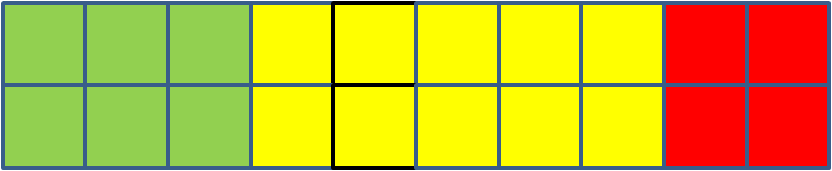What percentage is shaded red?

20

2

4

Look at the shading in this rectangle.What fraction is shaded yellow?

1/2

3 tenths

3/5

Look at the shading in this rectangle.What fraction is shaded yellow?

1/2

10 tenths

3/5

Look at the shading in this rectangle.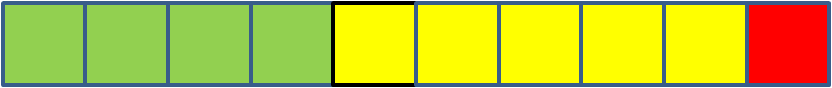What fraction is shaded green?

1/2

4/5

2/5

Look at the shading in this rectangle.What percentage is shaded green?

30

6

60

Look at the shading in this rectangle.What decimal fraction is shaded green?

0.3

0.6

6

Look at the shading in this rectangle.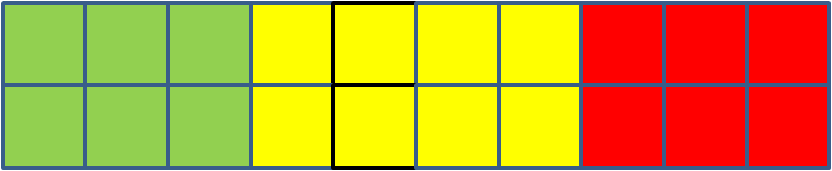What decimal fraction is shaded red?

0.6

0.3

6

Look at the shading in this rectangle.What fraction is shaded yellow?

1/2

0.8

2/5

Look at the shading in this rectangle.What percentage is shaded yellow?

8/10

40

80

• Question 1

Look at the shading in this rectangle.What decimal fraction is shaded yellow?

0.6
EDDIE SAYS
6 out of the ten squares in the fraction bar are shaded yellow, so 0.6 is the decimal fraction.
• Question 2

Look at the shading in this rectangle.What percentage is shaded red?

20
EDDIE SAYS
The whole is 20 squares and 4 are shaded red. 4 divided by 20 is 0.2 or 20 percent.
• Question 3

Look at the shading in this rectangle.What fraction is shaded yellow?

1/2
EDDIE SAYS
5/10 of the squares are shaded yellow, which reduces to 1/2.
• Question 4

Look at the shading in this rectangle.What fraction is shaded yellow?

1/2
EDDIE SAYS
10/20 of the squares are shaded yellow, which reduces to 1/2.
• Question 5

Look at the shading in this rectangle.What fraction is shaded green?

2/5
EDDIE SAYS
4 out of the ten squares is shaded green, or 4/10, which reduces to 2/5.
• Question 6

Look at the shading in this rectangle.What percentage is shaded green?

30
EDDIE SAYS
6/20 of the rectangle is shaded green, or 3/10, which is 30 percent.
• Question 7

Look at the shading in this rectangle.What decimal fraction is shaded green?

0.3
EDDIE SAYS
6/20, which reduces to 3/10, is shaded green. 3 divided by 10 is 0.3 which is the decimal.
• Question 8

Look at the shading in this rectangle.What decimal fraction is shaded red?

0.3
EDDIE SAYS
6/20 squares, which reduces to 3/10, are shaded red. 3 divided by 10 is 0.3 which is the decimal.
• Question 9

Look at the shading in this rectangle.What fraction is shaded yellow?

2/5
EDDIE SAYS
8/20 squares, which reduces to 2/5, is shaded yellow.
• Question 10

Look at the shading in this rectangle.What percentage is shaded yellow?

40
EDDIE SAYS
8/20 squares are shaded yellow, or 2/5, which is 0.4 or 40 percent.
---- OR ----

Sign up for a £1 trial so you can track and measure your child's progress on this activity.

### What is EdPlace?

We're your National Curriculum aligned online education content provider helping each child succeed in English, maths and science from year 1 to GCSE. With an EdPlace account you’ll be able to track and measure progress, helping each child achieve their best. We build confidence and attainment by personalising each child’s learning at a level that suits them.

Get startedStart your £1 trial today.
Subscribe from £10/month.